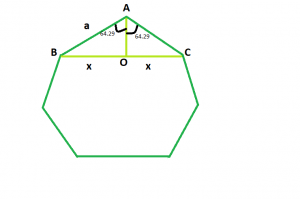# Diagonal of a Regular Heptagon

Given an integer a which is the side of a regular heptagon, the task is to find and print the length of its diagonal.Examples:

Input: a = 6
Output: 10.812

Input: a = 9
Output: 16.218

## Recommended: Please try your approach on {IDE} first, before moving on to the solution.

Approach: We know that the sum of interior angles of a polygon = (n – 2) * 180 where, n is the no. of sides in the polygon.
So, sum of interior angles of heptagon = 5 * 180 = 900 and each interior angle will be 128.58(Approx).
Now, we have to find BC = 2 * x. If we draw a perpendicular AO on BC, we will see that the perpendicular bisects BC in BO and OC, as triangles AOB and AOC are congruent to each other.
So, in triangle AOB, sin(64.29) = x / a i.e. x = 0.901 * a
Therefore, diagonal length will be 2 * x i.e. 1.802 * a.

Below is the implementation of the above approach:

## C++

 `// C++ Program to find the diagonal ` `// of a regular heptagon ` `#include ` `using` `namespace` `std; ` ` `  `// Function to return the diagonal ` `// of a regular heptagon ` `float` `heptdiagonal(``float` `a) ` `{ ` ` `  `    ``// Side cannot be negative ` `    ``if` `(a < 0) ` `        ``return` `-1; ` ` `  `    ``// Length of the diagonal ` `    ``float` `d = 1.802 * a; ` `    ``return` `d; ` `} ` ` `  `// Driver code ` `int` `main() ` `{ ` `    ``float` `a = 6; ` `    ``cout << heptdiagonal(a) << endl; ` `    ``return` `0; ` `} `

## Java

 `// Java program to find the diagonal of a regular heptagon ` `import` `java.util.*;  ` `import` `java.lang.*;  ` `import` `java.io.*;  ` ` `  `public` `class` `GFG {  ` ` `  `    ``// Function to return the diagonal of a regular heptagon ` `    ``static` `double` `heptdiagonal(``double` `a)  ` `    ``{  ` ` `  `//side cannot be negative ` `        ``if``(a<``0``) ` `        ``return` `-``1``; ` ` `  `        ``// length of the diagonal ` `        ``double` `d=``1.802``*a; ` `         `  `        ``return` `d;  ` `    ``}  ` ` `  `    ``// Driver code  ` `    ``public` `static` `void` `main(String[] args)  ` `    ``{  ` `        ``int` `a = ``6``;  ` `        ``System.out.println(heptdiagonal(a));  ` `    ``}  ` `}  `

## Python3

 `# Python3 Program to find the diagonal  ` `# of a regular heptagon  ` ` `  `# Function to return the diagonal  ` `# of a regular heptagon  ` `def` `heptdiagonal(a) : ` ` `  `    ``# Side cannot be negative  ` `    ``if` `(a < ``0``) : ` `        ``return` `-``1` ` `  `    ``# Length of the diagonal  ` `    ``d ``=` `1.802` `*` `a ` `     `  `    ``return` `round``(d, ``3``) ` ` `  `# Driver code  ` `if` `__name__ ``=``=` `"__main__"` `: ` ` `  `    ``a ``=` `6` `    ``print``(heptdiagonal(a))  ` ` `  `# This code is contributed by Ryuga `

## C#

 `// C# program to find the diagonal of a regular heptagon ` `using` `System; ` `public` `class` `GFG {  ` ` `  `    ``// Function to return the diagonal of a regular heptagon ` `    ``static` `double` `heptdiagonal(``double` `a)  ` `    ``{  ` ` `  `//side cannot be negative ` `        ``if``(a<0) ` `        ``return` `-1; ` ` `  `        ``// length of the diagonal ` `        ``double` `d=1.802*a; ` `         `  `        ``return` `d;  ` `    ``}  ` ` `  `    ``// Driver code  ` `    ``public` `static` `void` `Main()  ` `    ``{  ` `        ``int` `a = 6;  ` `        ``Console.WriteLine(heptdiagonal(a));  ` `    ``}  ` `} ``// This code is contributed by Mukul singh `

## PHP

 `

Output:

```10.812
```

Attention reader! Don’t stop learning now. Get hold of all the important DSA concepts with the DSA Self Paced Course at a student-friendly price and become industry ready.

My Personal Notes arrow_drop_upBudding Web DeveloperKeen learnerAverage CoderDancer&Social Activist

If you like GeeksforGeeks and would like to contribute, you can also write an article using contribute.geeksforgeeks.org or mail your article to contribute@geeksforgeeks.org. See your article appearing on the GeeksforGeeks main page and help other Geeks.

Please Improve this article if you find anything incorrect by clicking on the "Improve Article" button below.